# Plus Two Physics Chapter Wise Questions and Answers Chapter 2 Electric Potential and Capacitance

Students can Download Chapter 2 Electric Potential and Capacitance Questions and Answers, Plus Two Physics Chapter Wise Questions and Answers helps you to revise the complete Kerala State Syllabus and score more marks in your examinations.

## Kerala Plus Two Physics Chapter Wise Questions and Answers Chapter 2 Electric Potential and Capacitance

### Plus Two Physics Electric Potential and Capacitance NCERT Text Book Questions and Answers

Question 1.
Two charges 5 × 10-8C and -3 × 10-8C are located 16 cm apart. At what point on the line joining the two charges is the electric potential zero?
Take the potential at infinity to be zero.
Given q1 = 5 × 10-8C, r=16cm = 0.16m q2= -3 × 10-8C Let potential be zero at a distance × metre from positive charge q1.
∴ r1 = x meter
r2 = (0.16 – x) metre
S0 V = $$\frac{1}{4 \pi \varepsilon_{0}}\left[\frac{q_{1}}{r_{1}}+\frac{q_{2}}{r_{2}}\right]$$or 0.8 – 5x = 3x
or x = 0.1m = 10cm.Question 2.
A regular hexagonal of side 10cm has a charge 5mC at each of its vertices. Calculate the potential at the centre of the hexagon.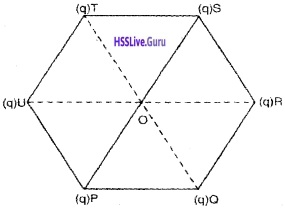From the figure, we have
OP = OQ = OR = OS = OT = OU
= r = 10cm = 0.1m
And given q = 5µC = 5 × 10-6C
∴ Potential at O due to all the charges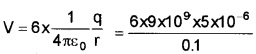= 2.7 × 106volt.

Question 3.
Two charges 2mC and -2mC are placed at points A and B 6cm apart.

1. Identify an equipotential surface of the system.
2. What is the direction of the electric field at every point on this surface?

1. The plane normal to AB and passing through its mid-point has zero potential everywhere hence the plane is equipotential.
2. Normal to the plane is the direction AB.Question 4.
A spherical conductor of radius 12cm has a charge of 1.6 × 10-7C distributed uniformly on its surface. What is the electric field

1. Inside the sphere.
2. Just outside the sphere.
3. At point 18cm from the centre of the sphere?

1. Zero

2.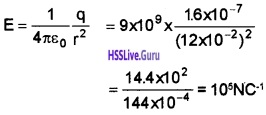3.Question 5.
A parallel plate capacitor with air between the plates has a capacitance of 8pF (1 pF=10-12F). What will be the capacitance if the distance between the plates is reduced by half, and the space between them is filled with a substance of dielectric constant s?
The capacitance of capacitor with air as dielectric is given by
C = $$\frac{\varepsilon_{0} A}{d}$$
Given C = 8pF = 8 × 10-12F …………(1)
If C1 is new capacitance when d1 = $$\frac{d}{2}$$ and space is filled with a substance of dielectric constant k=6.
Then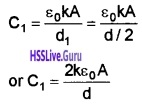Using Eq.(1)
C1 = 12 × 8 × 10-12F
or C1 = 96pF.Question 6.
Three capacitors each of capacitance 9pF are connected in series.

1. What is the total capacitance of the combination?
2. What is the potential difference across each capacitor, if the combination is connected to a 120 volt supply?

Given C1 = C2 = C3 = 9pF = 9 × 10-12F; V= 120 volt.
1. Total capacitance of the series combination is given by∴ C = 3 × 10-12F = 3pF.

2. Let q be the charge on each capacitor. Then, sum of the potential difference across their plates must be equal to 120 V.
ie. V1 + V2 + V1 = 120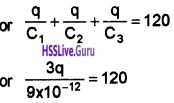or q = 360 × 10-12C
Since, all the capacitors are of same capacitance,### Plus Two Physics Electric Potential and Capacitance One Mark Questions and Answers

Question 1.
A hollowing metallic sphere of radius 10cm is charged such that potential on its surface is 80V. the potential at the center of the sphere would be.
(a) 80 V
(b) 800 V
(c) zero
(d) 8 V
(a) 80 V

Question 2.
When air is replaced by a dielectric medium of constant K, the maximum force of attraction between two charges separated by a distance.
(a) increases k times
(b) remains unchanged
(c) decreases k times
(d) increases k-1 times
(c) decreases k times
Explanation : Fm = $$\frac{\mathrm{F}_{0}}{\mathrm{K}}$$ i.e., Decrease K times.Question 3.
Charge q2 is at the center of a circular path with radius r. work done in carrying charged q1 once a round this equipotential path, would be.(c) zero

Question 4.
Pick out a vector quantity from the following
(i) Electric potential
(iii) Electric potential energy
(iv) Electric flux

Question 5.
“The surface of a charged conductor is an equipotential surface”. Comment on this statement.
In a charged conductor, at any point inside or at the surface, the potential is same. So the surface is equipotential.Question 6.
Pick the odd one out of the following
(i) eV
(ii) Volt
(iii) Mega Volt
(iv) Millivolt
(i) eV (eV is the unit of energy).

### Plus Two Physics Electric Potential and Capacitance Two Mark Questions and Answers

Question 1
Figure shows a parallel plate air capacitor of plate area of 100cm2 and separation 5mm. A potential difference of 300v is established between its plates by a battery.1. Calculate the capacitance and charge on the capacitor.
2. After disconnecting the battery, the space between the plate Js filled by ebonite (k=2.6). Then calculate the capacitance and charge on capacitor.

1.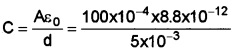= 17.6 pF
Q = CV= 17.6 × 10-12 × 300 = 5.2 × 10-9C

2. C = $$\frac{A K \varepsilon_{0}}{d}=\frac{K A \varepsilon_{0}}{d}$$
= 2.6 × 17.6 × 10-12 = 45.76pF.Question 2.
Fill in the blanks

1. 6.25 × 1018 electrons =………..C
2. 6.25 × 1018 eV =…………J

1. 1 coulomb
2. 1 J

Question 3.
Potential is a scalar quantity. Potential gradient is a vector quantity Negative of potential gradient is another vector quantity. It is
1.

• electric force
• electric flux
• electric field intensity
• none

2. What is magnitude and direction of above quantity in the case of a point charge

1. Electric field
2. Direction of electric field is outward for positive charge and inward for negative change

Question 4.

1. Dielectric strength of air is 3 × 106 V/m. What does it mean?
2. High power lines cannot be insulated. Why?

1. When electric field on air exceeds the 3 × 106 v/m, air becomes conductor and conduct electricity.
2. Insulator shows conducting property at high voltage.Question 5.

Write whether true or false

1. In a charged conductor charge reside inside and outside of the conductor
2. In a charged conductor net field is zero inside the conductor
3. Potential is Zero inside the conductor
4. The entire part of the conductor (including surface of the conductor) is at constant potential.

1. False
2. True
3. False
4. True

### Plus Two Physics Electric Potential and Capacitance Three Mark Questions and Answers

Question 1.
Match the following.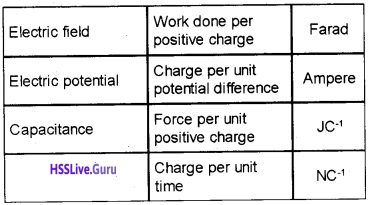1. Electric field – force per unit (+ve) charge -NC-1
2. Electric potential – Work done per (+ve) charge – JC-1
3. Capacitance – charge per unit potential difference -Farad.Question 2.
The data given below shows the variation of potential with charge during charging of a condenser.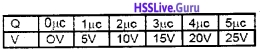1. Draw a graph with charge along the Y-axis and potential along the X-axis. Estimate capacity of the condenser from the graph.
2. Estimate the energy stored in the condenser from the graph.

1.2. Area enclosed by the graph represents total energy = 1/2 QV = 1/2 × 5 × 10-6 × 25
= 62.5 × 10-6 J

Question 3.
Many of the applications of capacitors depend on their ability to store energy.
1. In a charged capacitor energy is stored in the

• positively charged plate
• negatively charged plate
• electric field between the plates
• none of these

2. Draw a graph showing the variation of charge stored in a capacitor with its potential. How will you calculate the energy stored in the capacitor using the above graph?
1. Electric field between the plates

2.Area under the straight line graph gives the energy stored in the capacitor.Question 4.
A and B are two points in an electric field produced by q. To bring a unit +ve charge from a to A, 10 J work is needed. To bring the same charge from. A to B, 2J work is needed.
1. What is the p.d, between A and B?2. What are the potential at A and B?

1. 2v
2. potential at A, VA = 10v potential at B, VB = 12v.

Question 5.

1. 1 coulomb = 6.25 × 1018 electrons, 1 Joule =……..eV
2. What is meant by 1 eV
3. Which is bigger unit J or eV? Justify for your answer.

1. lev=1.6 × 10-19 J
∴ 1J = $$\frac{1}{1.6 \times 10^{-19}}$$ ev 1 J = 6.25 × 1018 ev.

2. 1 ev is the energy acquired by an electron, when it is accelerated through a potential difference of one volt.

3. Joule is bigger unit, 1J = 6.25 × 1018 eV.

Question 6.

1. Name the physical quantity which has its unit joule, coulomb-1. Is it a vector or a scalar?
2. Two plane sheets of charge densities +σ and -σ are kept in air as shown in figure. What are the electric field intensities at point A and B?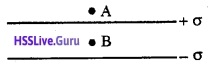1. Electric potetial. Scalar.

2. Electric field at A EA = 0
Electric field atB EB = $$\frac{\sigma}{\varepsilon_{0}}$$.Question 7.
You are given 3 capacitors of capacities 3 µf, 2 µf, 1 µf You have to make a capacitors of capacitance less than 1 µf.

1. How do you arrange the capacitors, show by a diagram?
2. Write an expression for effective capacitance of the above combination and verify the result.

1. Connect in series2.Question 8.
The arrangement of a capacitor is given below. The plate A is charged and the plate B is earthed, ‘d’ is the distance between two plates.
1. Write any one use of this capacitor.2. Obtain an expression for capacitance of this arrangement.

3. A capacitor is made of a flat plate of area A and second plate having a stair like structure as shown in figure. The width of each stair is d and 2d. Find the capacitance of this arrangement.1. To store electric charges

2. C = $$\frac{A \varepsilon_{0}}{d}$$

3. It is equivalent to two capacitors connected in parallel.
C = C1 + C2Question 9.
The dotted line indicate the surface such that they lie equi-distance from the charge ‘q’.

1. What is the name of this surface?
2. Write the properties of the surface.
3. Give a mathematical proof to any one of the properties.1. Equipotential surface.

2. properties of the surface:

• Direction of electric field is perpendicular to the equi potential surface,
• No work is done to move a charge from one point to another along the equi potential surface.

3. Work done = Potential difference × charge
= 0 × Charge = 0.Question 10.

1. What is meant by potential at a point?
2. Obtain an expression for potential at a point
3. A wire is bent in a circle of radius 10cm. It is given a charge of 250µC which spreads on it uniformly. What is the electric potential at the centre?

1. Potential at a point is the work done required bring a unit charge from infinity to that point without acceleration.

2. Potential due To A point charge: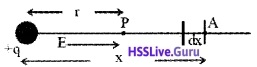Let P be a point at a distance Y from a charge +q. Let A be a point at a distance ‘x’ from q, and E is directed along PA. Consider a positive charge at A. Then the electric field intensity at ‘A’ is given by
$$E=\frac{1}{4 \pi \varepsilon_{0}} \frac{q}{x^{2}}$$
If this unit charge is moved (opposite to E} through a distance dx, the work done dw = – Edx
So the potential at ‘P’ is given byV = $$\frac{+q 1}{4 \pi \varepsilon_{0} r}$$ (since $$\frac{1}{\infty}$$ = 0).

3.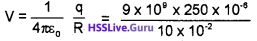= 2.25 × 107 v.

### Plus Two Physics Electric Potential and Capacitance Four Mark Questions and Answers

Question 1.

1. Write any one use of capacitor
2. Obtain an expression for capacitance of a parallel plate capacitor
3. The Capacity of a parallel plate capacitor becomes 10µF when air between the plates is replaced by a dielectric slab (k =2). What is the capacity of the capacitor with air in between the plates?

1. one use of capacitor:

• Capacitor is used to store electric charges
• It is used to prevent dc current.

2. Expression for capacitance of a capacitor:
Potential difference between two plates V= Ed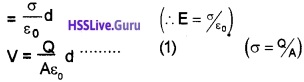Capacitance C of the parallel plate capacitor,C = $$\frac{\mathrm{A} \varepsilon_{0}}{\mathrm{d}}$$

3. C = 10µF
When dielectric slab is placed, New capacitance
C1 = KC
10 × 10-6 = 2 × C, C=5 × 10-6F.Question 2.
A charge of +5µC is placed in free space. P and Q are two points at 3mm and 5mm respectively from 5µC.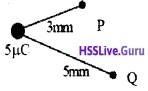1. The work done to bring a + IC charge from infinity to a point ‘P’ is called

• capacitance
• dielectric constant
• potential energy
• electric potential

2. Calculate the workdone in above process.

3. Calculate the workdone to move a +IC charge from ‘P’to ‘Q’.

1. Electric potential.

2. Work done w = VQ3. The potential energy at p,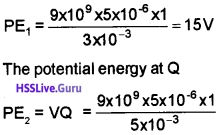PE2 = 9V
Work done to move IC from P to Q, W = PE2 – PE1
= 9 – 15
w = -6 J

Question 3.

1. “Electric field lines are always parallel to Equipotential surfaces.” Correct the statement if there is any mistake.
2. Draw the equipotential for a single positive point charge.

1. Electric field lines are always perpendicular to Equipotential surfaces.

2.Question 4.
E is the electric field intensity at any point in a uniform electric field.

1. What is meant by uniform electric field?
2. Represent a uniform electric field using lines of force.
3. Calculate energy stored per unit volume of the space if E=2V/m.

1. Region where magnitude and direction of electric field remain same.

2. lines of force are parallel and equidistant.3. Energy density = $$\frac{1}{2}$$ εo E2 = 1/2 × 8.85 × 1012 × 22
= 17.7 J/m3.Question 5.
In a charged capacitors energy is stored
1.

• in+ve plate
• In dielectric
• In electric field

2. Derive an expression for energy stored in a capacitor.

3. Draw a graph between energy and charge, in electric field

1. In electric field

2. If we supply a charge ‘dq’ to the capacitor, then work done can be written as,
dw = Vdq
dw = $$\frac{q}{c}$$ dq (since v = $$\frac{q}{c}$$)
∴ Total work done to charge the capacitor (from ‘0’ to ‘Q’) is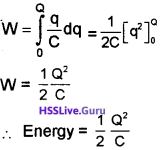### 3.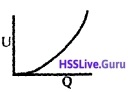Plus Two Physics Electric Potential and Capacitance Five Mark Questions and Answers

Question 1.1. Identify the device

• Moving coil Galvanometer
• Cyclotron
• Photovoltaic cell
• Van-de-Graft electrostatic generator

2. Explain the construction and working of the above device

3. What happens if the upper metal sphere is replaced by a cubical shaped metal? Explain.

1. Vandegraff generator

2. Van de graff generator:
Van de Graff generator is used to produce very high voltage.
Principle:
If two charged concentric hollow spheres are brought in to contact, charge will always flow from inner sphere to the outer sphere.
Construction and working: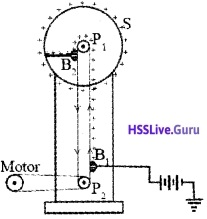The vande Graff generator consists of a large spherical metal shell, placed on an insulating stand. Let p1 and p2 be two pulleys. Pulley p1 is at the center of the spherical shell S. A belt is wound around two pulleys p1 and p2.

This belt is rotated by a motor. Positive charges are sprayed by belt. Brush B2transfer these charges to the spherical shell. This process is continued. Hence a very high voltage is produced on the sphere.

3. The charge density on the pointed edge is higher than flat surface. Hence charge will leak from the cubical shaped metal.Question 2.
The figure below shows a non polar dielectric slab placed in between the plates of an uncharged parallel plate capacitor.
Area of each plate = A, Distance, of separation be¬tween the plates = d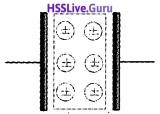1. If the dielectric slab is absent and the capacitor is charged to a surface charge density a, the electric field in between the plates is

• E = σεo
• E = $$\frac{\sigma^{2}}{\varepsilon_{0}}$$
• E = $$\frac{\sigma}{\varepsilon_{0}}$$
• E = σεo2

2. Redraw the given figure, which shows the align-ment of the nonpolar molecules, when the capacitor is charged.

3. Derive an expression for capacitance of the above capacitor with the dielectric slab in between the plates.
1. E = $$\frac{\sigma}{\varepsilon_{0}}$$

2.3. Effect of dielectric on capacitance: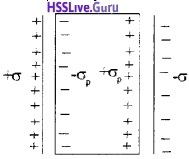Consider a capacitor of area A and charge densities +σ and -σ. Let d be the distance between the plates. If a dielectric slab is placed inside this capacitor, it undergoes polarization.

Let +σp and -σp be polarized charge densities due to polarization. Due to polarization electric field in between the plate becomes
E = $$\frac{\sigma}{\mathrm{K} \varepsilon_{0}}$$ …….(1)
The potential difference between the plates,
V = Ed ………..(2)
Sub (1) in (2)
V = $$\frac{\sigma}{\mathrm{K} \varepsilon_{0}}$$ d
Then the capacitance of capacitor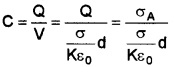C = $$\frac{A \varepsilon_{0} K}{d}$$
The product ε0K is the permittivity of the medium.Question 3.
Three capacitors C1, C2 and C3 are connected to a cell of emf V as shown in the figure.1. The arrangement of these three capacitors are called………….

• parallel combination
• series combination
• LCR combination
• c-c combination

2. Find the effective capacitance of the above combination.

3.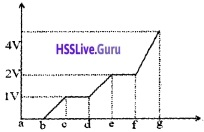The above graph shows the variation of potential in going from a to g. From the graph the relation among C1, C2 and C3 is

• C1 = C2 = C3
• 2C1 = 2C2 = C3
• C1 = C2 = 2C3
• C1 = C2 = C3

1. Series connection.

2. Capacitors in series:
Let three capacitors C1,C2 and C3 be connected in series to p.d of V. Let V1, V2 and V3 be the voltage across C1, C2 and C3.The applied voltage can be written as,
V=V1 + V2 + V3 ………..(1)
Charge ‘q’ is same as in all the capacitor. So,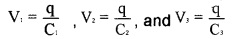Substituting these values in (1),If these capacitors are replaced by a equivalent capacitance ‘C’, then
V = $$\frac{q}{C}$$
Hence eq(2) can be written as
$$\frac{q}{C}=\frac{q}{C_{1}}+\frac{q}{C_{2}}+\frac{q}{C_{3}}$$
$$\frac{1}{C}=\frac{1}{C_{1}}+\frac{1}{C_{2}}+\frac{1}{C_{3}}$$
Effective capacitance is decreased by series combination.

3. From the graph, we get v1 = 1 v, v2 = 1 v, v3 = 2v.
This arrangement is series. Hence charge stored in each capacitor is same.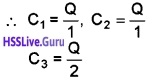C1: C2: C3
1: 1: 1/2
2: 2: 1
2C1: 2C2: C3Question 4.
Two metal plates X and Y of the area ‘A’ are separated by a distance ‘d’, charged + and – respectively.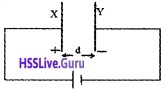1. This arrangement is called………….
2. The arrangement store energy in the………..(Magnetic field, Electric field, Electromagnetic field, Gravitational field)
3. Derive an expression for the energy stored in the arrangement.
4. When we increase separation between two plates by keeping V constant, what happens to total energy stored in the system.

1. Capacitor.

2. Electric field

3. If we supply a charge ‘dq’ to the capacitor, then work done can be written as,
dw = Vdq
dw = $$\frac{q}{c}$$ dq (since v = $$\frac{q}{c}$$)
∴ Total work done to charge the capacitor (from ‘0’ to ‘Q’) is4. When we increase the separation between two plates, capacitance (c) decreases. The energy in the capacitor U = 1/2 CV2 When c decreases, the energy decreases (because V is constant).

Question 5.
A combination of two conducting bodies separated by insulator can store electric charge
1. The above device is called………….

2. Derive on expression for capacitance of the device with air as medium.

3. If mica of dielectric constant Ks placed between the plates. What are its advantages? Explain action of mica in the case.
1. capacitor.

2. Potential difference between two plates V= EdCapacitance C of the parallel plate capacitor,3.

• When mica sheet is introduced, capacitance of capacitor increases
• Mica sheet will prevent electric breakdown.Question 6.
When a charged particle moves in an electric field, work is done on the particle.
1. Pick out a vector quantity from the following

• electric potential
• electric potential energy
• electric flux (1)

2. 5 J of work is done in moving a positive charge of 0.5C between two points. What is the potential difference between the points?

• 2.5V
• 10V
• 0.1V
• 5.5V (1)

3. Three-point electric charges q1 = 6µC, q2 = 4µC and q3 = -8µC are placed on the circumference of a circle of radius 1 m as shown in the figure. What is the value of the charge q4 placed on the circle if the potential at the centre of the circle is zero? (3)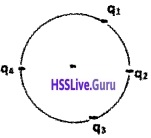2. 10V

3. Radius of the circle r = 1m
By the given condition
$$\frac{1}{4 \pi \varepsilon_{0}} \times \frac{1}{r}$$ (q1 + q2 + q3 + q4) = 0
(q1 + q2 + q3 + q4) = 0
(6 + 4 – 8 + q4) µC = 0
(2 + q4) µC = 0
q4 = -2µC.

Question 7.
Equipotential surface is a surface on which the electrical potential is the same at every point.
1. “Electric field lines are always parallel to Equipotential surfaces.” Correct the statement if there is any mistake. (1)

2. Draw the equipotential for a single positive point charge. (2)

3. A point charge +q is placed at the centre of a sphere of radius R. Another point charge +q is taken from a point A to another diametrically opposite point B on the surface of the sphere. Calculate the work done for this. (2)
1. Electric field lines are always perpendicular to Equipotential surfaces.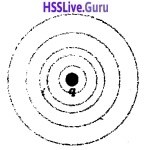2. Surface of the sphere is Equipotential in nature. So the potential difference between any points is zero. Since work done is the product of charge moving and the potential difference between the points, work done is equal to zero.Question 8.
Capacitance of a capacitor depends on the size and shape of the conductors and on the dielectric material between them.
1. Draw the symbol of a variable capacitor. (1)

2. The plates of a parallel plate capacitor are connected to an ideal voltmeter. What will happen to the reading of the voltmeter if the plates of the capacitor are brought closer to each other in an insulating medium? (2)

3. The plates of a parellel plate capacitor in vacuum are 5mm apart and 1.5m2 in area. A potential difference of 10kV is applied across the capacitor. Calculate
a. The capacitance
b. The charge on each plate.
c. magnitude of the intensity of electric field between the paltes.

1.2. Capacitance C = $$\frac{\varepsilon_{0} A}{d}$$
Potential difference V = $$\frac{q}{c}=\frac{q d}{\varepsilon_{0} A}$$
In an insulating medium charge remains the same,voltage is directly proportional to plate separation. Therefore, voltmeter reading decreases.

3.
a.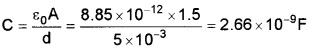b. Q = CV = 2.66 × 10-9 × 10 × 103 = 2.66 × 10-5 C.
c.Question 9.
Capacitors can be combined to obtain any desired capacitance in an application.
1. You are given two capacitors of capacitance 20µF each. Draw a diagram to show how you will connect these capacitors to get 40µF capacitance. (1)

2. Two capacitors C1 and C2 are connected in series.
a. Draw a diagram of the above combination. (1)
b. If C1 = 4µF, C2 = 6µF and C3 = 2.4µF and a potential difference of 100V is applied across the combination. Calculate the change stored in each capacitor.

1.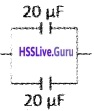2. Two capacitors C1 and C2 are connected in series:
a.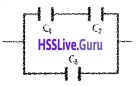b. Effective capacitance C1 anc C2
C12 = $$\frac{C_{1} C_{2}}{C_{1}+C_{2}}=\frac{4 \times 6}{4+6}$$ = 2.4µF
Potential difference across C12 = 100V
Charge stored on C12, q12 = C12 × 100
q12 = 2.4 × 10-6 × 100
q12 = 2.4 × 10-4 C
Charge on C1
q1 = 2.4 × 10-4C
Charge on C2
q2 = 2.4 × 10 -4C
Potential difference across C3 = 100V
Charge on C3
q3 = C3 × 100
q3 = 2.4 × 10-6 × 100
q3 = 2.4 × 10-4C.Question 10.
Many of the applications of capacitors depend on their ability to store energy.
1. In a charged capacitor energy is stored in the

• positively charged plate
• negatively charged plate
• electric field between the plates
• none of these (1)

2. Draw a graph showing the variation of charge stored in a capacitor with its potential. How will you calculate the energy stored in the capacitor using the above graph?

3. An electric flash lamp has 20 capacitors each of capacitance 5µF connected in parallel. The lamp is operated at 100V. If the energy stored in the combination is completely radiated out in a single flash, how much energy will be radiated in a flash? (2)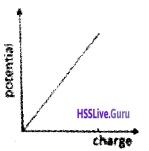Energy stored E = $$\frac{1}{2}$$ CV
E = $$\frac{1}{2}$$ × 100 × 10-6 × 1002 = 0.5 J